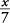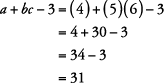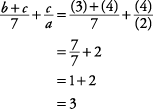## Variables and Algebraic Expressions

Before you start solving equations, you should have a basic understanding of variables, as well as translating and evaluating algebraic expressions.

#### Variables

A variable is a letter used to stand for a number. The letters xyzabcm, and n are probably the most commonly used variables. The letters e and i have special values in algebra and are usually not used as variables. The letter o is usually not used because it can be mistaken for 0 (zero).

#### Algebraic expressions

Variables are used to change verbal expressions into algebraic expressions, that is, expressions that are composed of letters that stand for numbers. Key words that can help you translate words into letters and numbers include:

• For addition: sum, more than, greater than, increase
• For subtraction: minus, less than, smaller than, decrease
• For multiplication: times, product, multiplied by, of
• For division: halve, divided by, ratio.

##### Example 1

Give the algebraic expression for each of the following.

1. the sum of a number and 5

2. the number minus 4

3. six times a number

4. x divided by 7

5. three more than the product of 2 and x

1. the sum of a number and 5: x + 5 or 5 + x

2. the number minus 4: x – 4

3. six times a number: 6 x

4. x divided by 7:or5. three more than the product of 2 and x: 2 x + 3

#### Evaluating expressions

To evaluate an expression, just replace the variables with grouping symbols, insert the values given for the variables, and do the arithmetic. Remember to follow the order of the operations: parentheses, exponents, multiplication/division, addition/subtraction.

##### Example 2

Evaluate each of the following.

1. x + 2 y if x = 2 and y = 5

2. a + bc – 3 if a = 4, b = 5, and c = 6

3. m 2 + 4 n + 1 if m = 3 and n = 2

4.if a = 2, b = 3, and c = 4

5. –5 xy + z if x = 6, y = 7, and z = 1

1.2.3.4.5.